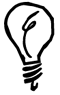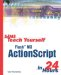# Strings and Numbers

 I l @ ve RuBoard

### Strings and Numbers

You can convert between strings and numbers and numbers and strings in a variety of ways. For instance, suppose that you have the user type in a number using an input field. Then you want to add 1 to that number. If num is the variable linked to that input field, you could try it like this:

` b = num + 1; `

If num contained "42", would the resulting value of "b" be 43? No. It would be "421." This is because "42" is a string with the characters "4" and "2" in it, not the number 42.

To make Flash see the "42" as a number, you use one of two functions to convert strings to numbers. The parseInt function takes a string and converts it to an integer. The parseFloat function takes a string and converts it into a floating point number.

So parseInt("42") returns the number 42. This function doesn't round, however, so parseInt("42.9") also resolves to 42. It just cuts the number off at the first non-digit.

If you use parseFloat("42.9") , you get 42.9. So, to round correctly, you could use Math.round(parseFloat("42.9")) . The parseFloat function also keeps the number as an integer if no floating point portion is needed. So parseFloat("42") resolves to 42. Unless you are strictly using integers, you might want to use parseFloat as your main conversion function.One cool feature of parseInt is that you can convert numbers that use other bases. For instance, if you wanted to convert hexadecimal numbers, base 16, to decimal numbers, just use 16 as the second parameter. So parseInt("FF",16) returns 255.

If you want to convert a number to a string, you need to use the toString function. This works a little differently than the parse functions because it is an operation performed on a number variable using dot syntax. Here is an example:

` a = 325; trace(a.toString() + 1); `

The result is "3251" as you might expect.You can use toString to convert a decimal number to a number of another base. This is the opposite of what the parseInt function can do, mentioned in the previous tip. If you supply a parameter with the base, you will get the appropriate results. So, (255).toString(16) returns "ff".

The semi-obsolete but convenient functions Number and String can also be used for conversions. These can be used to convert strings to numbers and vice versa. However, they are mostly present in Flash 6 as a backward compatibility measure for updating older Flash movies.

 I l @ ve RuBoardSams Teach Yourself Flash MX ActionScript in 24 Hours
ISBN: 0672323850
EAN: 2147483647
Year: 2002
Pages: 272

flylib.com © 2008-2017.
If you may any questions please contact us: flylib@qtcs.net